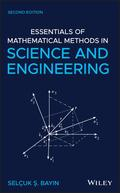John Wiley & Sons Essentials of Mathematical Methods in Science and EngineeringA comprehensive introduction to the multidisciplinary applications of mathematical methods, revised .. Product #: 978-1-119-58024-9 Regular price: \$142.06 \$142.06 Auf Lager

# Essentials of Mathematical Methods in Science and Engineering2. Auflage Februar 2020
960 Seiten, Hardcover
Lehrbuch

ISBN: 978-1-119-58024-9
John Wiley & Sons

Jetzt kaufen

### Preis: 152,00 €

Preis inkl. MwSt, zzgl. Versand

A comprehensive introduction to the multidisciplinary applications of mathematical methods, revised and updated

The second edition of Essentials of Mathematical Methods in Science and Engineering offers an introduction to the key mathematical concepts of advanced calculus, differential equations, complex analysis, and introductory mathematical physics for students in engineering and physics research. The book's approachable style is designed in a modular format with each chapter covering a subject thoroughly and thus can be read independently.

This updated second edition includes two new and extensive chapters that cover practical linear algebra and applications of linear algebra as well as a computer file that includes Matlab codes. To enhance understanding of the material presented, the text contains a collection of exercises at the end of each chapter. The author offers a coherent treatment of the topics with a style that makes the essential mathematical skills easily accessible to a multidisciplinary audience. This important text:

* Includes derivations with sufficient detail so that the reader can follow them without searching for results in other parts of the book

* Puts the emphasis on the analytic techniques

* Contains two new chapters that explore linear algebra and its applications

* Includes Matlab codes that the readers can use to practice with the methods introduced in the book

Written for students in science and engineering, this new edition of Essentials of Mathematical Methods in Science and Engineering maintains all the successful features of the first edition and includes new information.

1 Functional Analysis 1

2 Vector Analysis 55

3 Generalized Coordinates and Tensors 133

4 Determinants and Matrices 197

5 Linear Algebra 233

6 Practical Linear Algebra 293

7 Applications of Linear Algebra 355

8 Sequences and Series 425

9 Complex Numbers and Functions 453

10 Complex Analysis 491

11 Ordinary Differential Equations 527

12 Second-Order Differential Equations and Special Functions 589

13 Bessel's Equation and Bessel Functions 629

14 Partial Differential Equations and Separation of Variables 661

15 Fourier Series 705

16 Fourier and Laplace Transforms 727

17 Calculus of Variations 757

18 Probability Theory and Distributions 789

19 Information Theory 841
SELÇUK ^. BAYIN, PHD, is Professor in the Institute of Applied Mathematics at the Middle East Technical University in Ankara, Turkey. He has over thirty years of academic experience on the use of mathematical methods in physics courses, and his current research focuses on general relativity and cosmology. He is the author of the first and second editions of Mathematical Methods in Science and Engineering, and Essentials of Mathematical Methods in Science and Engineering, from Wiley.

S. S. Bayin, Middle East Technical University Ankara, Turkey